# Get the percentage for the sold product in Excel

In this article, we will learn about how to calculate the percentage for the sold product in excel.

Get the percentage for the sold product data in excel using a simple excel percent formula. Divide the sold product amount by the total amount of the product. You can find the percentage of anything using this method.
Syntax for the sales percentage formula:

Percent = Sold Amount / Total product

Generally, in mathematics we need to multiply the result by 100 to get the percentage value but whereas Excel considers decimal as a percentage value. You can change its formatting to percent by selecting the percentage option in Home > % (symbol under Number section) as shown in the below snapshot.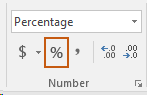Let’s understand this sales percentage formula used in the example shown below.
Let's say we sell something and that is recorded in the B column of the excel sheet. will use the percentage value formula to get the percentage value for the data.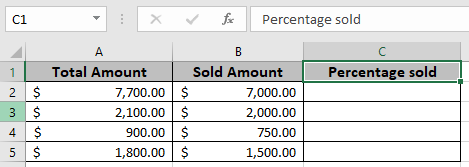Use the formula in D3 cell

= B2 / A2

Explanation : The sold amount will be divided by the total amount for a product.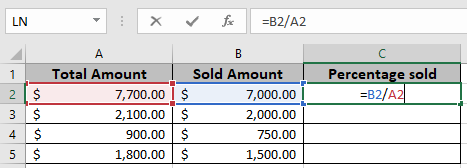Here the values to the function are given as cell reference.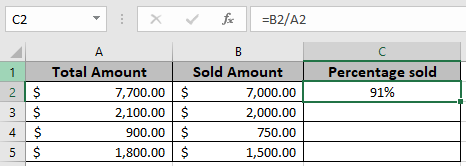As you can see in the above snapshot the first old Amount before increment is 91% .

Copy the formula in the remaining cells using Ctrl + D shortcut, to get the New Amount for the rest of the values in the table.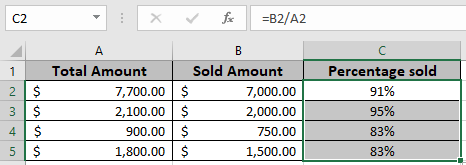In the above snapshot the mathematical formula is used to get the Percentage value for the sold product amount in excel.

Notes :

1. The function returns #VALUE error if any of the arguments are non numeric.
2. The function returns values for the negative as well as positive values.
3. Use the Increase by percentage formula to get the new or updated amount having percentage increase values.

So yeah mate, this is how to find the percent of something in excel. Explore more articles on Mathematical formulation like Increase by percentage and Profit margin percentage in Excel here. Mention your queries in the comment box below. We will help you with it.

Related Articles

Increase by percentage

Calculate Percentage of Total in Excel

Calculate Profit margin percentage

Popular Articles

Edit a dropdown list

If with conditional formatting

If with wildcards

Vlookup by date

Terms and Conditions of use

The applications/code on this site are distributed as is and without warranties or liability. In no event shall the owner of the copyrights, or the authors of the applications/code be liable for any loss of profit, any problems or any damage resulting from the use or evaluation of the applications/code.